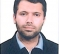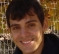## anti aliasing filterStarted by 6 years ago10 replieslatest reply 6 years ago236 views

Hi,

I want to sample a signal in 5 GHz and I assume there is a white noise of level m0.

Because I want design an antialiasing filter, when I sampled this signal this noise in higher frequency is replicated to desire frequency band.

How can I calculate total noise level  in desired band? or maximum noise level in desired band?

I mentioned  that noise in frequency 0-5GHz ,5-10GHz, 10-15GHz, …, 0.995-1THZ,1- 1.005THZ,  1.005-1.01THZ, … is replicated to 5GHz desired frequency.

Thanks.

[ - ]You need to look at integrated noise after the filter which is given by (m0)*(equivalent noise bandwidth of filter). For example: if you use a first order filter, the integrated noise is (m0)*(pi/2)*(w_3dB). Depending on the filter order, the equivalent noise bandwidth will change.

[ - ]but,

suppose: fs is frequence sample and fb is desired band and k is an integer value from minus infinity to plus infinity.

then we know when we sampled a signal noise in k*fs+fb frequency in original signal  is replicated to fb desired bandwidth, so noise is increased.

i want to know whats maximum value of noise after sampling and how calculted, or how can i approximate or evaluate noise  in desired band after sampling

[ - ]A white noise has infinite energy, if you do not filter the signal before sampling, you will have infinite noise energy. However, a true white noise do not exist in real-world. The thermal noise is not a real white noise, as its power density decays to zero at extremely high frequency. Also, your ADC, and all other components before him, has limited pass-band. So, you need to know the pass-band of your system in order to get an answer for your question.

Check this reference

[ - ]thanks for answer, your are right, but mathematically we impose white noise is extended to infinity. i want  a method to find total noise power to estimate the level of noise  to design anti aliasing filter ripple in  pass band and stop band

[ - ]I have never design an analog anti-aliasing filter. However, I know that If you are going to model your input as a white noise, you have to set a band limit to your filter, a frequency point where full attenuation is obtained after it.
[ - ]You are sampling at $$F_s$$.  Just from sampling, the signal at any frequency $$f$$ gets contributions from $$f \pm F_s, f \pm 2F_s, \cdots$$.

If the noise is truly white and uncorrelated, then the total noise power from aliasing will add -- not the noise amplitude.  So, let $$y(f)$$ be your signal after filtering and before sampling at frequency $$f$$.  The total noise power in your sampled signal is:

(sorry for the math-messing; this forum needs a preview mode)

$x_n \left ( f \right )^2 = \sum_{k=1}^\infty \left( y \left( f + k F_s \right)^2 + y \left( f - k F_s \right)^2 \right)$

[ - ]if i want design a 14 bit adc with supply voltage as 1v then total noise in pass band must be smaller than 1 LSB=(1/2^14).

if we assume a white noise then whats the max value of noise in pass band and stop band with attention to this  that noise in upper frequencies are replicated to our pass band after sampling.

[ - ]But you didn't.  What's missing?  I can't tell.

[ - ]hi

your formula, that you write is completely correct.but it does not determine  any way to calculate gain in stop band OR SLOPE of gain in stop band for  getting special noise level in desired band.

suppose thermal noise level  in 0-5GHZ is m0 and also to infinity frequency. we know noise in frequency 5ghz to infinity is replicated after sampling to desired band namely 0-5ghz.

[ - ]